Homology of Klein bottle

This article describes the value (and the process used to compute it) of some homotopy invariant(s) for a topological space or family of topological spaces. The invariant is homology and the topological space/family is Klein bottle
Get more specific information about Klein bottle | Get more computations of homology

Statement

The homology of the Klein bottle$K$ is given by: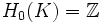$H_0(K) = \mathbb{Z}$

and:$H_1(K) = \mathbb{Z} \oplus \mathbb{Z}/2\mathbb{Z}$

All other homology groups are 0.

Related invariants

These are all invariants that can be computed in terms of the homology groups.

Invariant General description Description of value for Klein bottle
Betti numbers The$k^{th}$ Betti number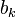$b_k$ is the rank of the$k^{th}$ homology group.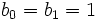$b_0 = b_1 = 1$,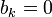$b_k = 0$ for$k > 1$
Poincare polynomial Generating polynomial for Betti numbers$1 + x$
Euler characteristic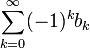$\sum_{k=0}^\infty (-1)^k b_k$ 0 -- consistent with the fact that its double cover is the 2-torus, which, being a nontrivial compact connected Lie group, has Euler characteristic zero, and the fact that Euler characteristic of covering space is product of degree of covering and Euler characteristic of base